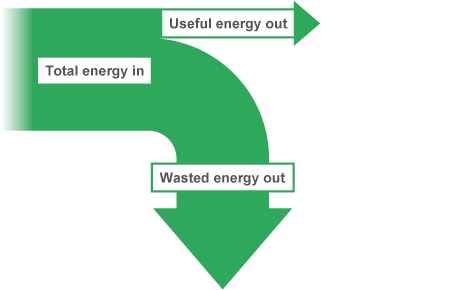# Energy and Work Topic

?
• Created by: Mum*
• Created on: 17-12-18 23:17

## Energy Transfers

Types of Energy:

• Chemical energy (Food)
• Thermal energy (Heat)
• Kinetic energy (Movement)
• Sound energy
• Light energy
• Gravitational (potential) energy
• Electrical energy
• Geothermal energy (Heat Underground)
• Elastic (potential) energy (Stored in springs e.g clocks)

Energy is not created or destroyed; it can only be transferred from one form to another

1 of 4

## Transferring energy

Energy is transferred by one of the following four types of energy transfer:

• Mechanical work – a force moving an object through a distance
• Electrical work – charges moving due to a potential difference
• Heating – due to temperature difference caused electrically or by chemical reaction
• Radiation – energy transferred as a wave, eg light, infrared, sound - light radiation & infrared radiation is emitted by the Sun.
• Doing 'work' is the scientific way of saying that energy has been transferred.
• For example, a grazing cow, a firing catapult and a boiling kettle are all doing 'work', as energy is being transferred.
2 of 4

## Sankey Diagrams

Sankey diagrams show the energy transfers in a system:• The total energy transferred into the system is shown as an arrow
• This arrow splits into narrower arrows showing other transfers

The width of the arrow is drawn to scale to show the amount of energy. Sankey diagrams show that the total energy transferred into a system is equal to the useful energy transferred plus the energy dissipated or 'wasted'. This confirms that energy is conserved.

3 of 4

## Energy and Work

• Whenever an object starts to move, a force must have been applied to it.
• When a force moves an object, energy is transferred and work is done
• When work is done moving the object, the supplied energy is transferred to the object so the work done is equal to the energy transferred.
• Both work and energy have unit of joules(J)
•  To calculate work done:

work done (J) = force (N) x distance moved (m)               J = N x m

• When a force, N, in newtons is applied through a
• Distance, measured in metre,m, (in direction of force)
4 of 4